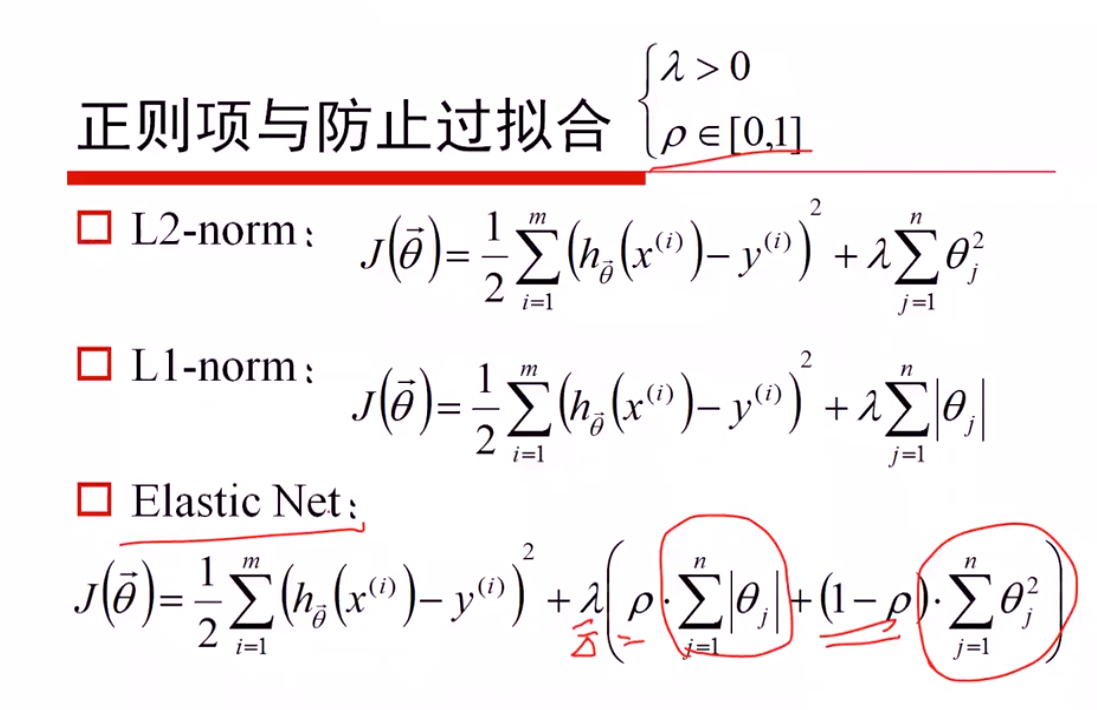# 机器学习—算法基础（六）线性回归与逻辑回归1038-茹同学

## 热门标签

### 线性回归

f

(

x

)

=

w

1

x

1

+

w

2

x

2

+

.

.

.

+

w

d

x

d

+

b

f(x)=w_1x_1+w_2x_2+…+w_dx_d+b

f(x)=w1x1+w2x2+...+wdxd+b

###### 梯度下降

w

=

w

0

+

α

f

w

0

w=w_0+/alpha/frac{/partial f}{/partial w_0}

w=w0+αw0f
f为损失函数，

α

/alpha

α为学习速率需要手动指定。

f

w

0

/frac{/partial f}{/partial w_0}

w0f表示方向，需要沿着这个函数下降的方向找，最后能找最低点。

#### 过拟合与欠拟合（线性回归）

1、原始特征过多，存在一些嘈杂特征，模型过于复杂

1、进行特征选择，消除关联性大的特征
2、交叉验证
3、正则化

1、原始特征较少

1、进行特征选择，消除关联性大的特征
2、交叉验证

##### 正则化

机器学习把L1和L2叫正则化，统计学领域叫惩罚项，数学领域叫范数。


R

(

f

)

=

1

N

i

=

1

n

L

(

y

i

,

f

(

x

i

)

)

+

λ

J

(

f

)

R(f)=/frac1N/sum_{i=1}^n{L(y_i,f(x_i))}+/lambda J(f)

R(f)=N1i=1nL(yi,f(xi))+λJ(f)

• 第一项即损失函数的表示
• 后一项中的J(f)就表示模型的复杂度 ，它是定义在假设空间F上的泛函（通常是指一种定义域为函数，而值域为实数的“函数”。换句话说，就是从函数组成的一个向量空间到实数的一个映射。也就是说它的输入为函数，而输出为实数，来自维基百科），模型f越复杂，复杂度J(f)就越大；反之，模型越简单，复杂度J(f)就越小。也就是说，复杂度表示了对复杂模型的惩罚。λ≥0是系数，用以权衡经验风险和模型复杂度。结构风险小的模型往往对训练数据以及位置的测试数据都有较好的预测。

##### ElasticNet回归### 逻辑回归

##### sigmod函数

Sigmoid函数是一个有着优美S形曲线的数学函数，在逻辑回归、人工神经网络中有着广泛的应用。Sigmoid函数的数学形式是：

f

(

x

)

=

1

1

+

e

x

f/left(x/right)=/frac1{1+e^{-x}}

f(x)=1+ex1

##### 逻辑回归公式

h

θ

(

x

)

=

g

(

θ

T

x

)

=

1

1

+

e

θ

T

x

h_/theta/left(x/right)=g/left(/theta^Tx/right)=/frac1{1+e^{-/theta^Tx}}

hθ(x)=g(θTx)=1+eθTx1

##### 逻辑回归的损失函数、优化

cos

t

(

h

θ

(

x

)

,

y

)

=

i

=

1

m

y

i

log

(

h

θ

(

x

)

)

(

1

y

i

)

log

(

1

h

θ

(

x

)

)

/cos t/left(h_/theta/left(x/right),y/right)=/sum_{i=1}^m-y_i/log/left(h_/theta/left(x/right)/right)-/left(1-y_i/right)/log/left(1-h_/theta/left(x/right)/right)

cost(hθ(x),y)=i=1myilog(hθ(x))(1yi)log(1hθ(x))

##### 逻辑回归的参数估计### SoftMax分类____ 判别模型 生成含义
____ 逻辑回归 朴素贝叶斯

### ROC曲线### AUC面积

AUC面积为ROC曲线的覆盖面积，其覆盖面积的大小表示分类器的好坏。

• AUC = 1，是完美分类器。
• AUC = [0.85, 0.95], 效果很好
• AUC = 0.5，跟随机猜测一样（例：丢铜板），模型没有预测价值。
• AUC < 0.5，比随机猜测还差.
##### AR自回归模型

θ

/theta

θ表示自回归系数。

x

(

t

)

=

θ

1

x

(

t

1

)

+

θ

2

x

(

t

2

)

+

+

θ

m

x

(

m

1

)

+

u

(

t

)

x(t)=/theta_1x(t-1)+/theta_2x(t-2)+/cdots+/theta_mx(m-1)+u(t)

x(t)=θ1x(t1)+θ2x(t2)++θmx(m1)+u(t)

##### MA

MA(Moving Average Model)移动平均模型。通过将一段时间的白噪声序列进行加权和，可以得到移动平均方程。表示为MA（q）

θ

/theta

θ表示移动回归系数，ut表示不同时间点的白噪声。

x

(

t

)

=

θ

1

u

(

t

1

)

+

θ

2

u

(

t

2

)

+

+

θ

m

u

(

m

1

)

+

u

(

t

)

x(t)=/theta_1u(t-1)+/theta_2u(t-2)+/cdots+/theta_mu(m-1)+u(t)

x(t)=θ1u(t1)+θ2u(t2)++θmu(m1)+u(t)

##### ARMA

ARMA(Auto Regressive and Moving Average Model)自回归移动平均模型，自回归移动平均模型是自回归和移动平均模型两部分组成。所以可以表示为ARMA(p,q)。p是自回归阶数，q是移动平均阶数。

x

(

t

)

=

θ

1

x

(

t

1

)

+

θ

2

x

(

t

2

)

+

+

θ

m

x

(

m

1

)

+

θ

1

u

(

t

1

)

+

θ

2

u

(

t

2

)

+

+

θ

m

u

(

m

1

)

+

u

(

t

)

x(t)=/theta_1x(t-1)+/theta_2x(t-2)+/cdots+/theta_mx(m-1)+/theta_1u(t-1)+/theta_2u(t-2)+/cdots+/theta_mu(m-1)+u(t)

x(t)=θ1x(t1)+θ2x(t2)++θmx(m1)+θ1u(t1)+θ2u(t2)++θmu(m1)+u(t)

##### 时间序列分析##### ARIMA

ARIMA(Auto Regressive Integrate Moving Average Model)差分自回归移动平均模型。ARIMA模型是为了解决在ARMA基础上，非平稳系列的模型。也是基于平稳的时间序列的或者差分化后是稳定的，另外前面的几种模型都可以看作ARIMA的某种特殊形式。表示为ARIMA(p, d, q)。p为自回归阶数，q为移动平均阶数，d为时间成为平稳时所做的差分次数，也就是Integrate单词的在这里的意思。

### 评论 抢沙发### 觉得文章有用就打赏一下文章作者

#### 支付宝扫一扫打赏#### 微信扫一扫打赏Vieu3.3主题Q Q 登 录